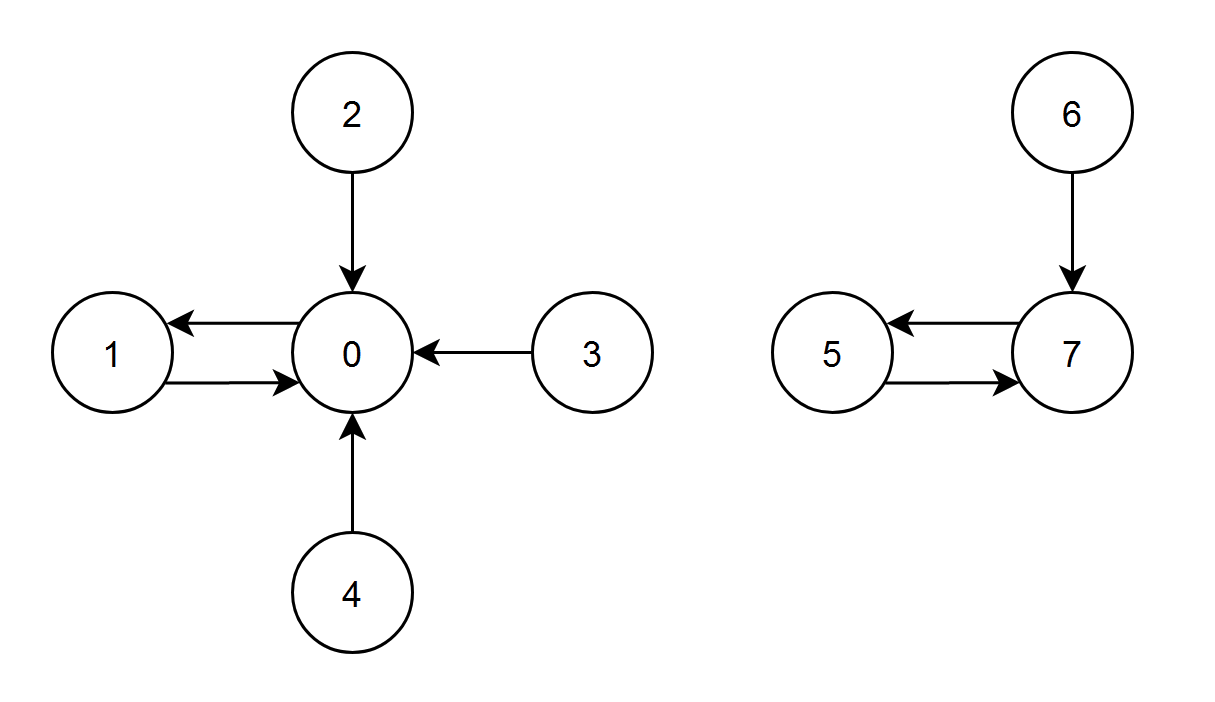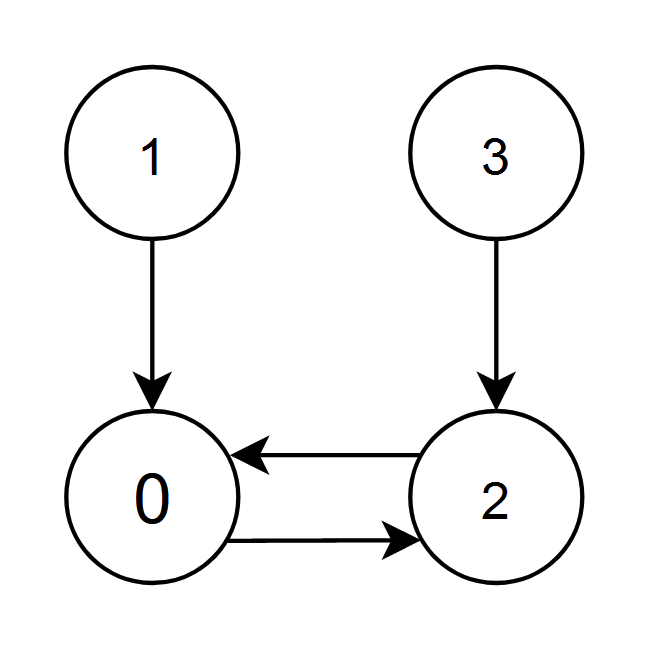##### Welcome to Subscribe On Youtube

Formatted question description: https://leetcode.ca/all/2374.html

# 2374. Node With Highest Edge Score

• Difficulty: Medium.
• Related Topics: Hash Table, Graph.
• Similar Questions: Two Sum, Sort Characters By Frequency, Sort Array by Increasing Frequency.

## Problem

You are given a directed graph with n nodes labeled from 0 to n - 1, where each node has exactly one outgoing edge.

The graph is represented by a given 0-indexed integer array edges of length n, where edges[i] indicates that there is a directed edge from node i to node edges[i].

The edge score of a node i is defined as the sum of the labels of all the nodes that have an edge pointing to i.

Return the node with the highest **edge score. If multiple nodes have the same **edge score, return the node with the smallest index.

Example 1:Input: edges = [1,0,0,0,0,7,7,5]
Output: 7
Explanation:
- The nodes 1, 2, 3 and 4 have an edge pointing to node 0. The edge score of node 0 is 1 + 2 + 3 + 4 = 10.
- The node 0 has an edge pointing to node 1. The edge score of node 1 is 0.
- The node 7 has an edge pointing to node 5. The edge score of node 5 is 7.
- The nodes 5 and 6 have an edge pointing to node 7. The edge score of node 7 is 5 + 6 = 11.
Node 7 has the highest edge score so return 7.


Example 2:Input: edges = [2,0,0,2]
Output: 0
Explanation:
- The nodes 1 and 2 have an edge pointing to node 0. The edge score of node 0 is 1 + 2 = 3.
- The nodes 0 and 3 have an edge pointing to node 2. The edge score of node 2 is 0 + 3 = 3.
Nodes 0 and 2 both have an edge score of 3. Since node 0 has a smaller index, we return 0.


Constraints:

• n == edges.length

• 2 <= n <= 105

• 0 <= edges[i] < n

• edges[i] != i

## Solution (Java, C++, Python)

• class Solution {
public int edgeScore(int[] edges) {
int n = edges.length;
int[] score = new int[n];
int maxScore = 0;
int node = 0;
for (int i = 0; i < n; i++) {
score[edges[i]] += i;
if (score[edges[i]] >= maxScore) {
if (score[edges[i]] == maxScore) {
node = Math.min(node, edges[i]);
} else {
node = edges[i];
}
maxScore = score[edges[i]];
}
}
return node;
}
}

############

class Solution {
public int edgeScore(int[] edges) {
int n = edges.length;
long[] cnt = new long[n];
for (int i = 0; i < n; ++i) {
cnt[edges[i]] += i;
}
int ans = 0;
for (int i = 0; i < n; ++i) {
if (cnt[ans] < cnt[i]) {
ans = i;
}
}
return ans;
}
}

• class Solution:
def edgeScore(self, edges: List[int]) -> int:
cnt = Counter()
for i, v in enumerate(edges):
cnt[v] += i
ans = 0
for i in range(len(edges)):
if cnt[ans] < cnt[i]:
ans = i
return ans

############

# 2374. Node With Highest Edge Score
# https://leetcode.com/problems/node-with-highest-edge-score/

class Solution:
def edgeScore(self, edges: List[int]) -> int:
n = len(edges)
scores =  * n

for node, out in enumerate(edges):
scores[out] += node

res = maxScore = -1

for node, score in enumerate(scores):
if score > maxScore:
maxScore = score
res = node

return res


• class Solution {
public:
int edgeScore(vector<int>& edges) {
int n = edges.size();
vector<long long> cnt(n);
for (int i = 0; i < n; ++i) {
cnt[edges[i]] += i;
}
int ans = 0;
for (int i = 0; i < n; ++i) {
if (cnt[ans] < cnt[i]) {
ans = i;
}
}
return ans;
}
};

• func edgeScore(edges []int) int {
n := len(edges)
cnt := make([]int, n)
for i, v := range edges {
cnt[v] += i
}
ans := 0
for i, v := range cnt {
if cnt[ans] < v {
ans = i
}
}
return ans
}

• function edgeScore(edges: number[]): number {
const n = edges.length;
const sum = new Array(n).fill(0);
for (let i = 0; i < n; i++) {
sum[edges[i]] += i;
}
let res = 0;
for (let i = 0; i < n; i++) {
if (sum[res] < sum[i]) {
res = i;
}
}
return res;
}



Explain:

nope.

Complexity:

• Time complexity : O(n).
• Space complexity : O(n).